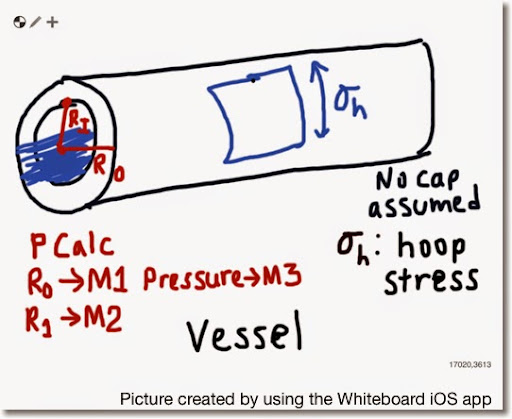## Sunday, May 25, 2014

### PCalc and HP 50g: Internal Pressure in a VesselPCalc and HP 50g: Internal Pressure in a Vessel

I = inner radius of the vessel
O = outer radius of the vessel
P = pressure of the water flow
σh = hoop stress

σh = P * (O^2 + I^2)/(O^2 - I^2)

Source: Goswami, Indramil Ph.D. P.E. "All In One Civil Engineering PE Breadth and Depth Exam Guide" 2nd Edition. McGraw Hill: 2012

PCalc: Pressure In A Vessel

Store the following:
I → M1
O → M2
P → M3

Decimal Mode
Set R1 To M1
R1 To Power of 2
Set R2 To M2
R2 To Power of 2
Set X To R1
Add R2 To X
Set R0 To R1
Subtract R2 From R0
Divide X By R0
Multiply X By M3

HP 50g: VESSEL

<< "Inner Radius" PROMPT
"Pressure" PROMPT
→ I O P
<< O SQ I SQ DUP2
+ UNROT - / P * →NUM
"σh" →TAG >> >>

Example:
O = 3/8 in = .375 in
I = 3/32 in = .09375 in
P = 10 lb/(s*in^2)

Result:
σh ≈ 11.3333 lb/(s*in^2)

- Eddie -